Previous: 8.4.4 Boundary Conditions Up: 8. Finite Difference Wigner Next: 9. Stochastic Methods and

# 8.5 Remarks on Implementation

The discretization is only sparse in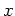, it is dense in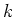. That means for a fixedall pairs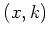and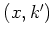couple in the matrix and give full blocks in.

The need to use an equispaced grid inis a performance killer. For resonant tunneling diodes one gets sharp resonances which have to be resolved by a fine enough-mesh. Although the equation is sparse in, the terms for potential and for scattering give full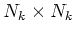blocks in the matrix. For self-consistent simulations one usually chooses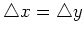where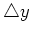is given by 8.15. Then the completeness condition shows the following unpleasant feature: if one refines the mesh everywhere inand also doubles the number of points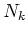, then the resolution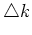inis unchanged. The-mesh is only extended towards infinity, but not denser. The new-points are introduced in external areas where the density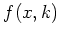is very low and accuracy inis not increased. Hence in a large part of the simulation domain the density is very low as depicted in Figure 8.1. In this case one has to increase the number of points inby a factor ofto achieve double resolution.If the nodes are ordered properly, the overall sparsity structure of the matrix is a banded block matrix with bandwidth of an order like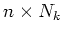where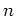depends on the chosen discretization for the free term (that is, a small number, for example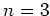). This Wigner system can be conveniently solved with a (sparse) Gaussian elimination solver. Note that the bandwidth for the Wigner system is approximately of the same order as for the matrix stemming from a two dimensional diffusion problem (Laplace equation). So with respect to the use of sparse Gaussian solvers, the Wigner equation is no worse than a completely" sparse problem. But a two-dimensional diffusion problem can conveniently be solved by iterative solvers, while the low overall sparsity (full blocks) of the Wigner system makes this slow.

Numerical costs for Gaussian elimination applied on amatrix block are of the order of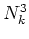. The number of such blocks in the system matrix is proportional to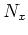. Hence the costs are of the order of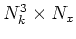which is approximately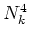. Even in one spatial dimension this sets a tight practical limit on the feasible size of the mesh. The Wigner function method is compared with the QTBM in Section 10.1 .